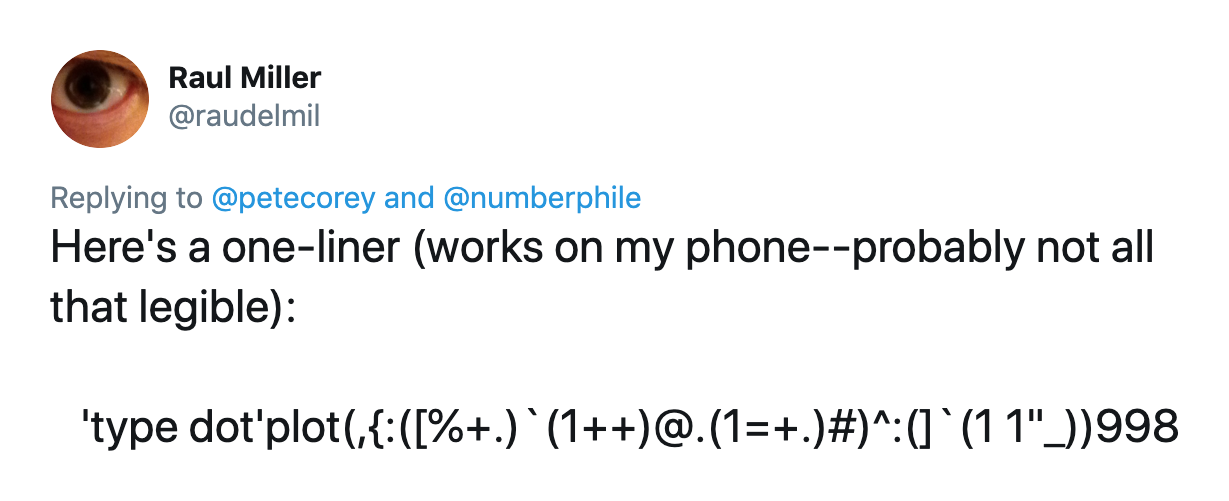Numberphile recently posted a video about an interesting recursive function called “Fly Straight, Dammit!” which, when plotted, initially seems chaotic, but after six hundred thirty eight iterations, instantly stabilizes.

This sounds like a perfect opportunity to flex our J muscles and plot this function ourselves!

## An Imperative Solution

The simplest approach to plotting our “Fly Straight, Dammit!” graph using the J programming language is to approach things imperatively:

``````a =: monad define
if. y < 2 do.
1
else.
py =. a y - 1
gcd =. y +. py
if. 1 = gcd do.
1 + y + py
else.
py % gcd
end.
end.
)
``````

We’ve defined our `a` monadic verb to return `1` if we pass in a “base case” value of `0` or `1`. Otherwise, we recursively execute `a` on `y - 1` to get our `py`, or “previous `y`”. Next, we check if the `gcd` of `y` and `py` equals `1`. If it does, we return `1 + y + py`. Otherwise, we return `py` divided by `gcd`.

This kind of solution shouldn’t look too foreign to anyone.

Let’s plot values of `a` to verify our solution:

``````require 'plot'
'type dot' plot a"0 i. 1000
``````This works, but it’s very slow. We know that our recursive calls are doing a lot of duplicated work. If we could memoize the results of our calls to `a`, we could save quite a bit of time. Thankfully, memoizing a verb in J is as simple as adding `M.` to the verb’s declaration:

``````a =: monad define M.
...
)
``````

Now our imperative solution is much faster.

## Using Forks and Hooks

While our initial solution works and is fast, it’s not taking advantage of what makes J a unique and interesting language. Let’s try to change that.

The meat of our solution is computing values in two cases. In the case when `y` and `py` have a greatest common divisor equal to `1`, we’re computing `1` plus `y` plus `py`. Our imperative, right to left implementation of this computation looks like this:

``````1 + y + py
``````

We could also write this as a “monadic noun fork” that basically reads as “`1` plus the result of `x` plus `y`:

``````a_a =: 1 + +
``````

Similarly, when we encounter the case where the greatest common divisor between `y` and `py` is greater than `1`, we want to compute `py` divided by that `gcd`. This can be written as a “dyadic fork”:

``````a_b =: [ % +.
``````

We can read this fork as “`x` divided by the greatest common divisor of `x` and `y`.”

Now that we’ve written our two computations as tacit verbs, we can use the “agenda” verb (`@.`) to decide which one to use based on the current situation:

``````a_a =: 1 + +
a_b =: [ % +.

if. y < 2 do.
1
else.
py =. a y - 1
has_gcd =. 1 = y +. py
py (a_b ` a_a @. has_gcd) y
end.
)
``````

If `has_gcd` is `0`, or “false”, we’ll return the result of `py a_b y`. Otherwise, if `has_gcd` is `1`, we’ll return the result of `py a_a y`.

## More Agenda

We can elaborate on the idea of using agenda to conditionally pick the verb we want to apply to help simplify out base case check.

First, let’s define our base case and recursive case as verbs that we can combine into a gerund. Our base case is simple. We just want to return `1`:

``````base_case =: 1:
``````

Our recursive case is just the (memoized) `else` block from our previous example:

``````recursive_case =: monad define M.
py =. a y - 1
has_gcd =. 1 = y +. py
py (a_b ` a_a @. has_gcd) y
)
``````

Our function, `a` wants to conditionally apply either `base_case` or `recursive_case`, depending on whether `y` is greater or less than one. We can write that using agenda like so:

``````a =: base_case ` recursive_case @. (1&<)
``````

And because our `base_case` verb is so simple, we can just inline it to clean things up:

``````a_a =: 1 + +
a_b =: [ % +.

py =. a y - 1
has_gcd =. 1 = y +. py
py (a_b ` a_a @. has_gcd) y
)

a =: 1: ` recursive_case @. (1&<)
``````

Using agenda to build conditionals and pseudo-“case statements” can be a powerful tool for incorporating conditionals into J programs.

## Going Further

It’s conceivable that you might want to implement a tacit version of our `recursive_case`. Unfortunately, my J-fu isn’t strong enough to tackle that and come up with a sane solution.

That said, Raul Miller came up with a one-line solution (on his phone) and posted it on Twitter. Raul’s J-fu is strong.